# Frequency stability of the beat-note between two free-running DFB lasers

2020-02-17

In this post, we drive two DFB lasers with two CTL101-2-B-200 laser controllers and observe the interference between the two lasers in the RF domain. Both lasers are narrow linewidth diodes from Eblana photonics EP1550-0-NLW-B26-100FM, operating around 1550 nm. We set the current of each laser at approximately 180 mA and tune the temperature of each laser so that the output wavelength reads 1550.000 nm on a Yokogawa AQ6150B wavelength meter.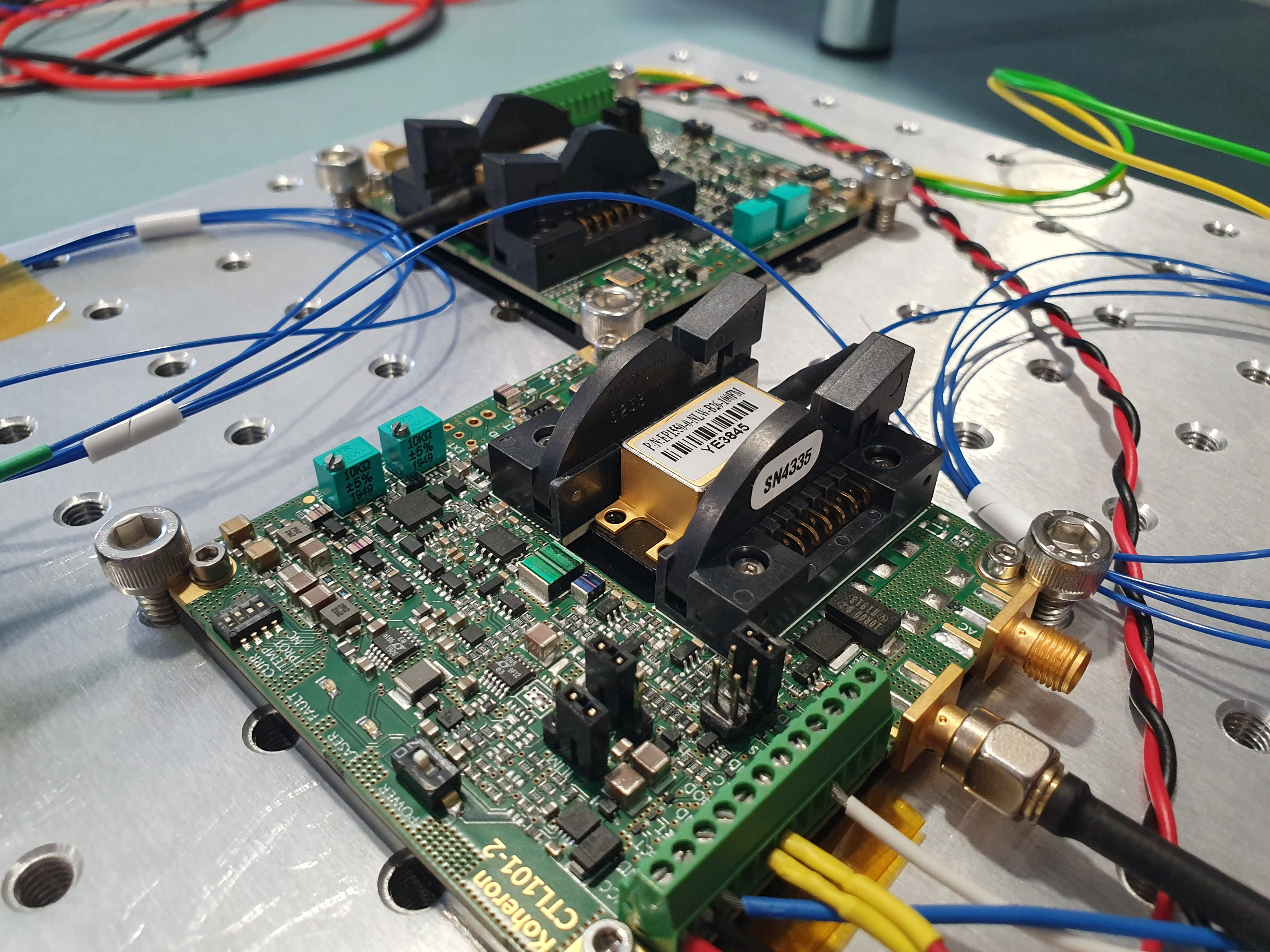We then use a 50/50 fiber coupler to combine the two lasers, perform the optical-to-electrical conversion with a Koheron PD100 photodetector and observe the RF spectrum in real-time on the Koheron ALPHA250 acquisition board: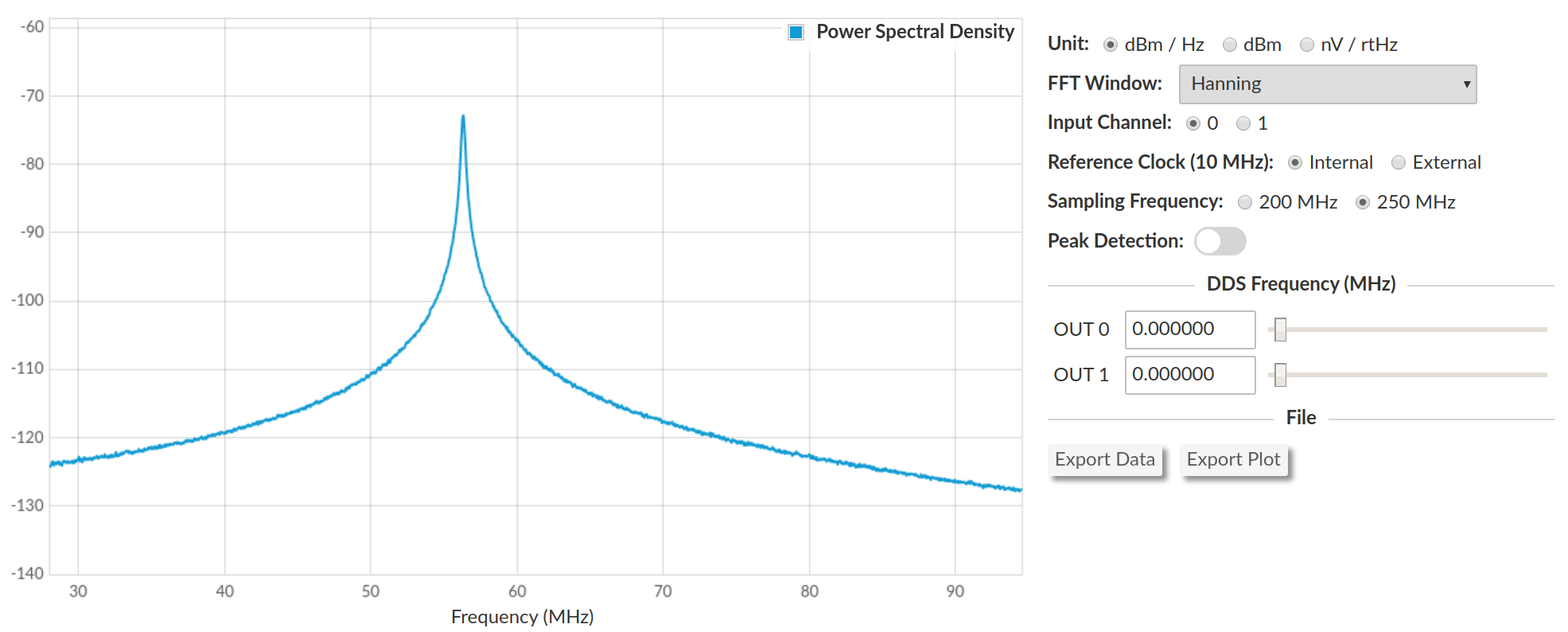As expected, the spectrum has a nice Lorentzian shape. The fluctuation of the peak frequency over time gives a good indication of the overall stability (current + temperature) of the CTL101 controllers. We wrote a Python script to measure the peak frequency every 50 ms during 24 hours: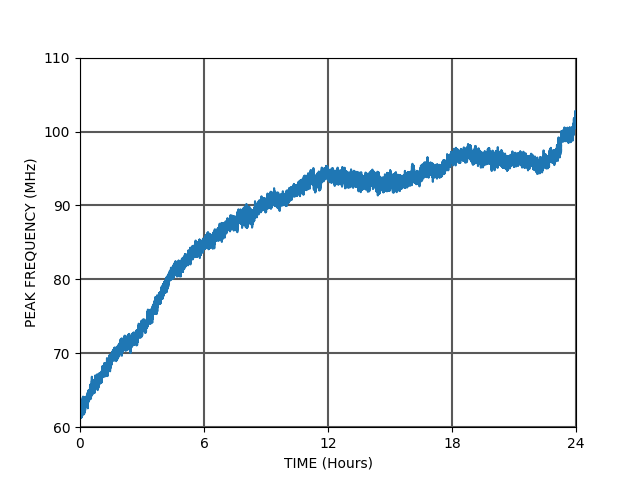We observe a frequency drift of about 30 MHz over the first 12 hours. The figure below shows the evolution of the peak frequency over the first minute: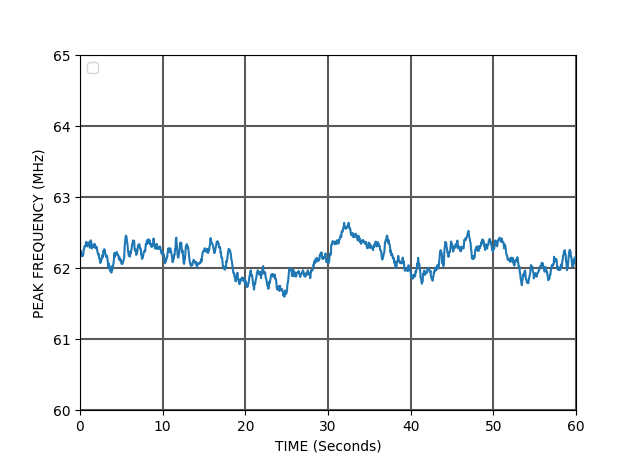When dealing with non stationary signals such as clock frequencies, the Allan deviation is a useful statistical metric: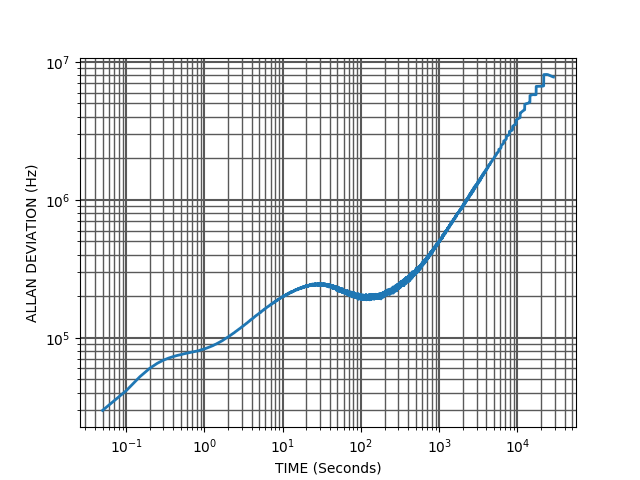The lasers used have a tunability of 2 nm, which corresponds to a frequency of 250 GHz. Since the Allan deviation below one minute is lower than 250 kHz, we can roughly say that the CTL101 controllers have an overall 1 ppm stability on a one minute timescale.

If the frequency variations of each laser were statistically independent, the Allan variance of the frequency of one laser would be the Allan variance of the beat note divided by two. However, the frequency variations of each laser are probably correlated because the two controllers, which have similar temperature dependencies, undergo almost the same ambient temperature variations.